An object’s Centre of Gravity or CG is its balancing point, if an object was supported at this point then it would be in equilibrium and not move.

Another way to think of centre of gravity is that it’s the point from which you can consider all of an object’s mass to act for the purposes of mechanical calculations.

Centre of gravity is widely used in engineering. It is particularly useful for designing objects (bridges, buildings etc) and also in predicting how an object will move when acted on by a force, whether that is gravity or another force.

## How Do We Measure CG in AutoCAD 2D?

In the AutoCAD 2D we can measure it in the following ways:

• In the case of a regular shape, we can find it by locating its centre
• In the case of a composite shape, we can find it either by locating it’s centres or by using AutoCAD commands.
• In the case of an irregular shape, we can find it using AutoCAD commands

We will mainly use the following commands in addition to the other AutoCAD commands.

• Pdmode
• UCS
• Point
• ‘Cal
• Region
• Massprop etc.

## Finding A Regular Shape’s Centre of Gravity

I will start this with the easiest of all the geometries, circle.

## Circle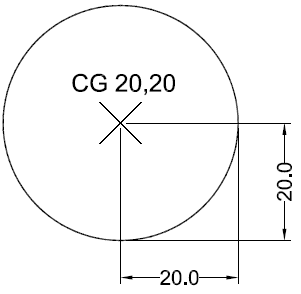The above is a 40 mm diameter circular object, it’s centre of gravity is it’s geometric centre so at 20,20.

## Rectangle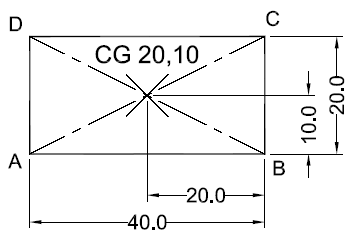To find the CG of a rectangular object we need to again find it’s centre. We do this by, drawing the diagonals AC & BD then measuring the intersecting point of the diagonals from A and B. This gives us the CG of the rectangle as 20, 10.

## Triangle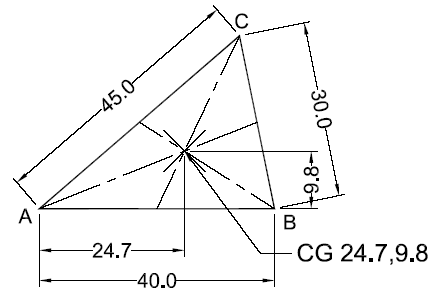We can find out the CG of a triangular object by drawing lines from A to the midpoint of BC, from B to midpoint of AC, and from the C to the midpoint of AB. Then, finding the intersecting point of the three diagonals and measuring its displacement from point A. The CG of the triangle is 24.7, 9.8

## Finding A Composite Shape’s Centre Of Gravity

Type A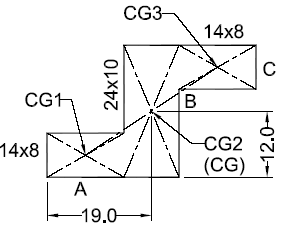The object above is a symmetrical composite object, which is effectively composed of three rectangular objects. Two (A and C) have the dimensions 14 x 8 and one central object (B) has dimensions 24 x 10.

To find out its CG, we need to draw the diagonals for each of part A, B, and C then find the intersecting points of the above diagonals which gives us the centres of gravity of each of the three ‘pieces’ of the shape. These are shown by CG1, CG2, CG3 on the diagram. Connect CG1and CG3 and you will find that it also intersects with CG2.

Now, the CG of the above composite object is CG2. Measure the CG from point A, it is 19, 12

Type B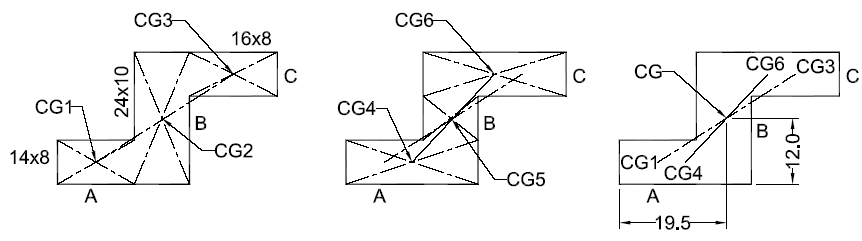To find out the CG of the above asymmetrical composite object, we need to perform following operations;

• Draw the diagonals for ‘pieces A, B, and C as is shown in the 1st figure
• Finding the intersecting points of the diagonals i.e. CG1, CG2, CG3
• Connecting CG1and CG3. As it doesn’t intersect with CG2 we have not yet found the object’s centre of gravity.
• Keep the line CG1CG3
• Change the way that we choose to split up the object. As you can see in figure 2 we now have two sections that are 14 x 18 and one that is 8 x 10.
• Draw the diagonals of new ‘pieces’ A, B, and C as you can see in figure 2.
• Find the intersecting points of the above diagonals i.e. CG4, CG5, CG6
• Connect the CG4 and CG6. Again it doesn’t not intersect with CG 5 and so we have not found the CG yet.
• However as the line CG4CG6 intersects with line CG1CG3 (see the 3rd figure) we have now found the centre of gravity, it is where the two lines cross.
• Measure the CG from the lower-left corner of part A, i.e. 19.5, 12

## Finding The Centre of Gravity of An Irregular Shape

Type A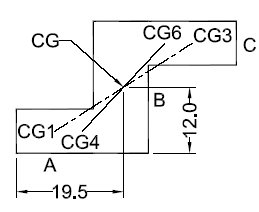We found the CG for the above object above. To illustrate our new method clearly we’ll first show it on this shape. We will convert the above geometry into a region.

• Command: region (press Enter)

Select objects: At this prompt, select the closed geometry and press enter.

1 loop extracted.

1 Region created.

• Command: UCS (press Enter)

Specify origin of UCS or [Face/NAmed/OBject/Previous/View/World/X/Y/Z/ZAxis] :

At this prompt, select the lower left corner point of part A (from where we will measure the CG); see, the UCS moves instantly from its original position to this corner point.

Specify point on X-axis or : At this prompt, press Enter to accept it.

• Command: MASSPROP (press Enter)

Select objects: At this prompt, select the above region and press enter.

AutoCAD will now give us a list of parameters of above object in a window as below: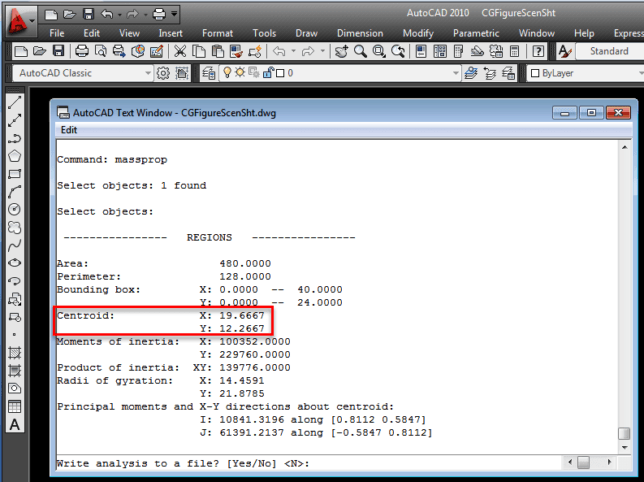In above window, we can find the Centroid or CG (marked red area) in terms of X & Y values i.e. 19.6667 & 12.2667.

Write analysis to a file? [Yes/No] : At this prompt, press Enter for No. (Or, if you need the data, press F2 and copy the data then paste it in any word doc.). Now, dimension the above centroid (CG) from the lower left corner of part A.

Types B and C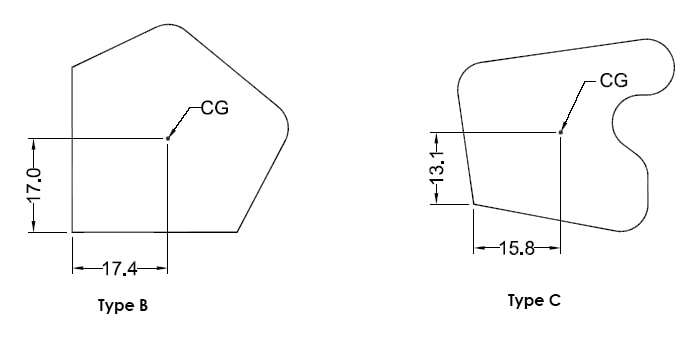Geometrically finding the CG of complex or irregular shapes is either extremely time-consuming or not practically possible. Using AutoCAD we can find CG of irregular shapes shown above very quickly, using following steps:

• Convert the area into a region
• Set the UCS at the lower left corner point of the object
• Find the Centroid (CG) through the ‘Massprop’ command (i.e. 17.4, 17.0 or 15.8, 13.1 ) which gives the dimensions of the CG from the lower left corner of the object.